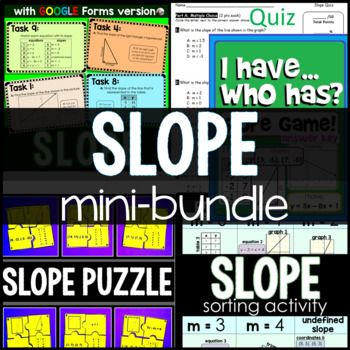DID YOU KNOW:
Seamlessly assign resources as digital activities

Learn how in 5 minutes with a tutorial resource. Try it Now

Learn More# SLOPE mini-bundle8th - 9th
Subjects
Standards
Resource Type
Formats Included
• Zip
\$12.50
Bundle
List Price:
\$15.50
You Save:
\$3.00
\$12.50
Bundle
List Price:
\$15.50
You Save:
\$3.00This bundle contains one or more resources with Google apps (e.g. docs, slides, etc.).

#### Also included in

1. This big bundle includes both print and digital activities to reinforce and review slope. It includes a slope partner scavenger hunt, a whole class scavenger hunt, task cards, puzzles, a slope tree to decorate your classroom bulletin board, a slope challenge PowerPoint game, a slope digital math esc
\$29.50
\$44.50
Save \$15.00
2. This bundle includes 24 activities for teaching, practicing and reviewing slope and algebraic functions. Also included is a set of Linear Equations Flippables for the slope, slope-intercept and point-slope equations. Many of the activities in this bundle have been updated with digital versions. Beca
\$58.00
\$88.50
Save \$30.50

### Description

Slope comes in many forms. This 4-part bundle includes 3 slope activities (slope sorting activity, slope task cards, slope game) and a slope quiz to summarize student learning. The 4 parts are linked below:

Slope Sorting Activity

Slope comes in many forms. This activity helps students identify slope when it is presented in different ways. Students work in pairs to cut, sort then paste different representations of slope (tables, graphs, equations, coordinate pairs and triangles) into 6 categories: slope 0 through slope 4 and undefined slope. The preview is a completed activity printed on bright paper.

Slope comes in many forms. Students work either independently or together to find slopes given: graphs, right triangles, tables of data, equations, and coordinate pairs (8.F.A.2). On each of the 10 task cards is an additional question, which asks students to find the equations of the lines represented in the data (8.F.B.4). You may choose to have your students work only to find slope or also to find these equations. A student answer sheet is included as well as an answer key.

I Have... Who Has...? Slope

Through a game of "I Have...Who Has?", students have fun identifying slope presented in different forms (tables, graphs, coordinate pairs, equations, and right triangles). The set includes 30 cards. Each student in a large class can be given one card, or in a smaller class more than one card can be given to each student.

The game starts with one student reading the "Who has?" portion of his or her card, which is then answered by the student in the class who has the answer. For example, one student may ask "Who has a table showing a slope of -2?". The student in the class with this table on his or her card says that they have the table and then goes on to ask the class their "Who has?" question.

The game can be started from any card as the game will always loop back around to the card that started the game. An answer key is included.

Slope Puzzle Activity

n this activity, students cut out 24 puzzle pieces with different representations of slope (graphs, tables, equations, coordinate pairs, right triangles) and fit same-slope pieces together. Each completed puzzle has 4 pieces with a graph, table, coordinate pair and either a right triangle or an equation on each of the pieces. When completed, each activity makes 6 completed puzzles with puzzles for slope 0, undefined slope, and slopes 1 through 4. Puzzle pieces are not matched on the sheets so that students can be in charge of cutting them out. The preview is a completed activity printed on colorful paper (actual puzzle pieces are larger, 2 completed puzzles will fit on 1 piece of paper).

Editable Slope Quiz

This quiz covers: finding slope from graphs, from tables, from sets of coordinate pairs and solving for y to make y=mx+b equations through a series of multiple choice and short answer questions. This quiz is meant to accompany Slope Sorting Activity: Identifying Slope in Different Forms as a way to differentiate your curriculum and assessment. Quiz is editable (in Word 7) and an answer key is is included.

You may also like:

Slope Activities Bundle

Total Pages
Included
Teaching Duration
N/A
Report this Resource to TpT
Reported resources will be reviewed by our team. Report this resource to let us know if this resource violates TpT’s content guidelines.

### Standards

to see state-specific standards (only available in the US).
Calculate and interpret the average rate of change of a function (presented symbolically or as a table) over a specified interval. Estimate the rate of change from a graph.
Construct a function to model a linear relationship between two quantities. Determine the rate of change and initial value of the function from a description of a relationship or from two (𝘹, 𝘺) values, including reading these from a table or from a graph. Interpret the rate of change and initial value of a linear function in terms of the situation it models, and in terms of its graph or a table of values.
Compare properties of two functions each represented in a different way (algebraically, graphically, numerically in tables, or by verbal descriptions). For example, given a linear function represented by a table of values and a linear function represented by an algebraic expression, determine which function has the greater rate of change.